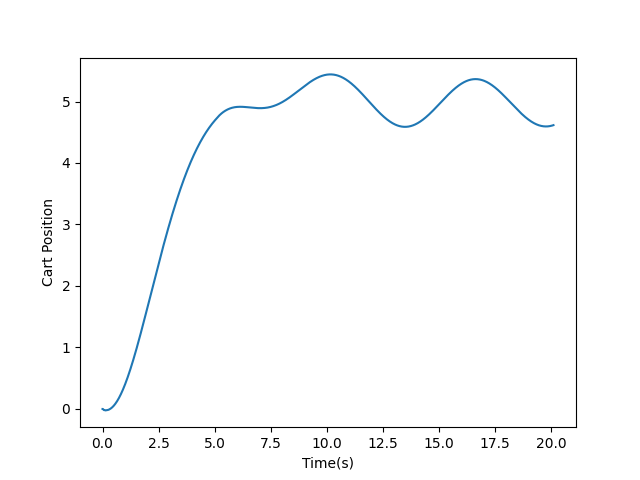# Run Real-Time Application by Using Python Script

This example shows how to call Simulink® Real-Time™ functions from a Python® script to build a real-time application from a model, load and run the application, tune parameter values, and capture signal data.

### Set Up MATLAB Session for Python

To set up your MATLAB® session for this example:

1. Open MATLAB and install the MATLAB engine for Python. For more information, see Call MATLAB from Python.

2. Convert the MATLAB session into a shared session. In the Command Window, type `matlab.engine.shareEngine`. For more information, see `matlab.engine.shareEngine`.

### Set Up Files and Run Python Script

To set up files and run the Python script:

1. Copy model `slrt_ex_pendulum_100Hz` to a working folder.

2. Copy Python script `CallingSlrealtimeFromPython.py` to the same working folder.

3. Open an operating system Command Prompt window and make the working folder the current folder for this window.

4. To run the Python script, at the command prompt, type:

`py CallingSlrealtimeFromPython.py -m [.slx file path] -t [target name]`

5. The Python script builds the model, runs the real-time application, and generates a plot of the captured signal data for cart position.### Line-by-Line Description of Python Script

The Python script uses the Simulink Real-Time function API to run the real-time application and capture the signal data.

Observe these points in the script:

• To call MATLAB commands from Python, import the `matlab.engine` module.

`import matlab.engine`
• Class `modelManagement` handles open and load of the Simulink model, then it builds the model.

`class modelManagement():`
• Class `targetManagement` is responsible for handling interactions with the `slrealtime.Target` object in MATLAB. For example, this class loads the application, starts the application, stops the application, gets and sets parameter values, and captures signal data.

`class targetManagement():`
• In the main function, the function tries to find all the active, shared MATLAB sessions and connects to the first one. If there is no session, the function opens a new MATLAB session.

```engs = matlab.engine.find_matlab() if not engs: eng = matlab.engine.start_matlab() else: eng = matlab.engine.connect_matlab(engs)```
• In the main function, the function instantiates a `modelManagement` object and builds the model.

```mm = modelManagement(eng, modelFilePath) appPath = mm.buildModel()```
• In the main function, the function instantiates a `targetManagement` object for the given target name.

`tg = targetManagement(eng, targetName)`
• In the main function, the function loads the application on the target computer.

`tg.load(appPath)`
• In the main function, the function creates a `slrealtime.Instrument` object in MATLAB, adds a signal for cart position to the instrument object, adds this instrument object to the target object, and enables the `BufferData` mode. The live-streamed signal is saved in memory and waits for retrieval. For more information about buffered data mode, see `getBufferedData`.

```blockPaths = ['slrt_ex_pendulum_100Hz/Pendulum'] portNumbers =  tg.captureSignals(appPath, blockPaths, portNumbers)```
• In the main function, the function starts to run the application on the target computer.

`tg.start()`
• In the main function, the function waits for 5 seconds, then sets the Wave Control block parameter `Value` to 2. This setting causes the cart to move in a sinusoidal pattern. The value is read again to make sure that the parameter value has been successfully updated.

```tg.setparam('slrt_ex_pendulum_100Hz/Wave Control','Value',2) newValue = tg.getparam('slrt_ex_pendulum_100Hz/Wave Control','Value') assert newValue == 2```
• In the main function, the function waits for 15 seconds and stops the application.

```time.sleep(15) tg.stop()```
• In the main function, the function retrieves the signal data and transfers the data back to Python.

`[t, data] = tg.getCapturedSignals('slrt_ex_pendulum_100Hz/Pendulum',1)`
• In the main function, the function removes the previously added instrument object from the target object, leaving the target object in a clean state.

`tg.removeInstrument()`
• In the main function, the function plots the captured signal data against the time. In the resulting plot, you can see that the cart position is stabilized at 5 around 5 seconds, and then the cart starts to move in a sinusoidal pattern as expected after 5 seconds.

```plt.plot(t, data) plt.xlabel('Time(s)') plt.ylabel('Cart Position') plt.show()```